Question

# 2) (i) State the converse of the Alternate Interior Angle Theorem in Neutral Geometry. (ii) Prove...

2) (i) State the converse of the Alternate Interior Angle Theorem in Neutral Geometry.

(ii) Prove that if the converse of the Alternate Interior Angle Theorem is true, then all

triangles have zero defect.

[Hint: For an arbitrary triangle, ABC, draw a line through C parallel to side AB. Justify

why you can do this.]

5) Consider the following statements:

I: If two triangles are congruent, then they have equal defect.

II: If two triangles are similar, then they have equal defect.

III: If two triangles have equal defect, then they are similar.

IV: If two triangles have equal defect, then they are congruent.

Identify which statements can be proved in Neutral Geometry and which cannot be

proved in Neutral Geometry. If a statement can be proved, provide a proof. If it cannot

be proved, state why not.

3) Let us build a geometry, S, using the three axioms of incidence geometry with one

Incidence Axiom 1:

For every point P and every point Q (P and Q not equal), there

exists a unique line,l

, incident with P and Q.

Incidence Axiom 2:

For every line l

there exist at least two distinct points incident with l.

Incidence Axiom 3:

There exist (at least) three distinct points with the property that no

line is incident with all three of them.

Axiom 4

: There exist at most four points.

(i) The elliptic parallel property is true, false or independent of the axioms of S.

Which one is it? Prove your answer. . [Recall that the elliptic parallel

property says: Given a line l and a point P not on l, there exists no line through P parallel to l.]

(ii) The Euclidean parallel property is true, false or independent of the axioms of S.

Which one is it? Prove your answer. [Recall that the Euclidean parallel

property says: Given a line l

and a point P not on

l, there exists

exactly one line through P parallel to l.]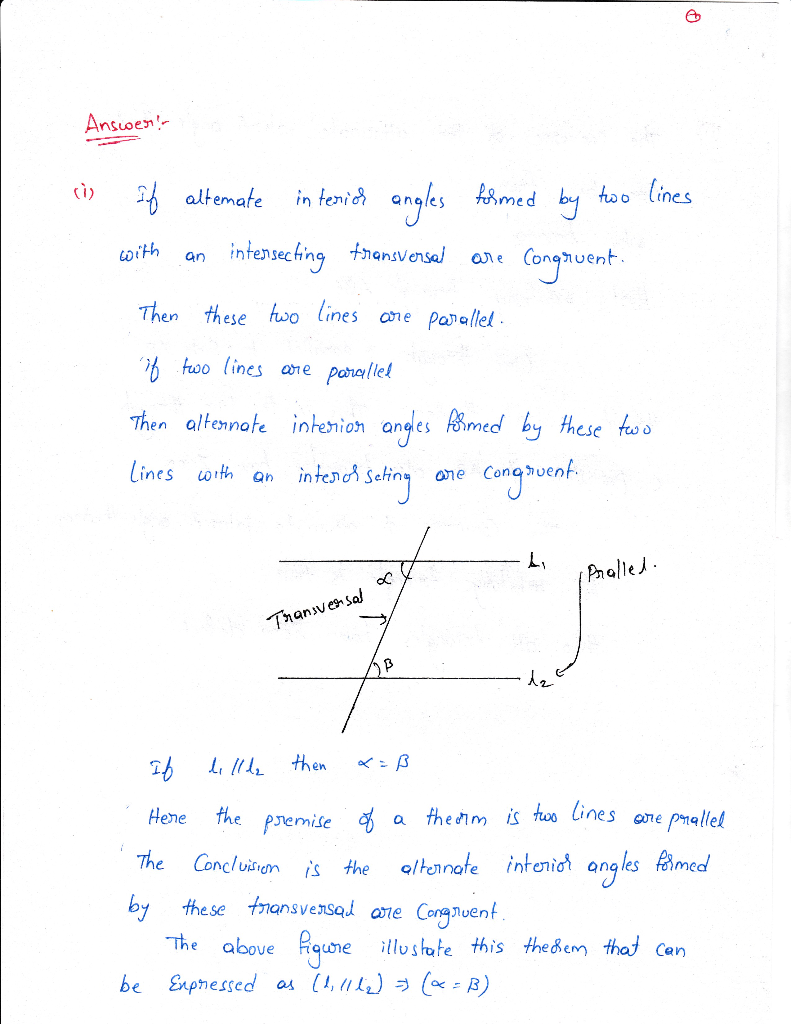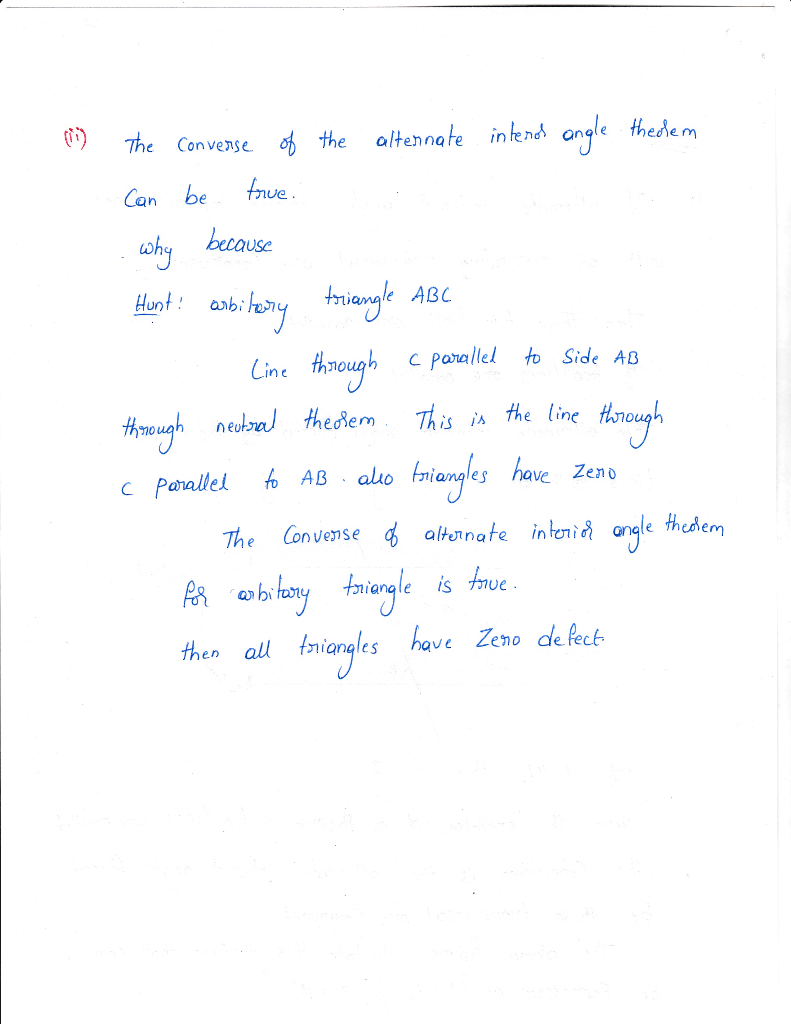#### Earn Coins

Coins can be redeemed for fabulous gifts.

Similar Homework Help Questions
• ### 3) Let us build a geometry, S, using the three axioms of incidence geometry with one additional a...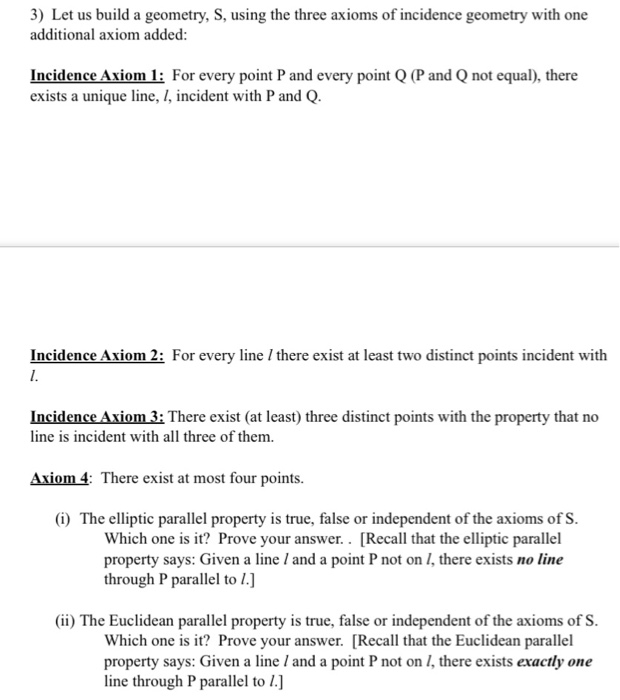3) Let us build a geometry, S, using the three axioms of incidence geometry with one additional axiom added: Incidence Axiom l: For every point P and every point Q (P and Q not equal), there exists a unique line, I, incident with P and Q. Incidence Axiom 2: For every line / there exist at least two distinct points incident with Incidence Axiom3: There exist (at least) three distinct points with the property that no line is incident with...

• ### 3) Let us build a geometry, S, using the three axioms of incidence geometry with one...3) Let us build a geometry, S, using the three axioms of incidence geometry with one additional axiom added: Incidence Axiom l: For every point P and every point Q (P and Q not equal), there exists a unique line, I, incident with P and Q. Incidence Axiom 2: For every line / there exist at least two distinct points incident with Incidence Axiom3: There exist (at least) three distinct points with the property that no line is incident with...

• ### 12. In Theorem 4.1 it was proved in neutral geometry that if alternate in- terior angles result in hyperbolic geometry...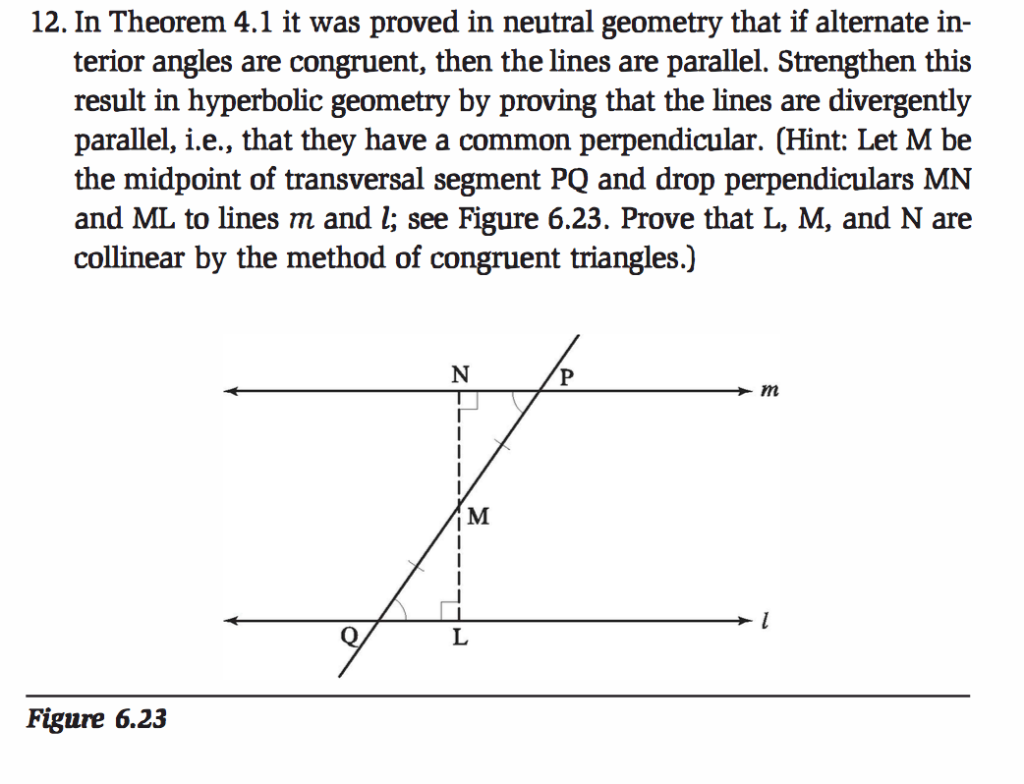12. In Theorem 4.1 it was proved in neutral geometry that if alternate in- terior angles result in hyperbolic geometry by proving that the lines are parallel, i.e., that they have a common perpendicular. (Hint: Let M be the midpoint of transversal segment PQ and drop perpendiculars MN and ML to lines m and l; see parallel. Strengthen this divergently are congruent, then the lines are Figure 6.23. Prove that L, M, and N are collinear by the method of...

• ### Part III (3 pts) For cach of the property statement below, determine which geometry would BEST xhoi given property (choose only one!). Please use A. for Euclidean geometry, B. for hypere geometry...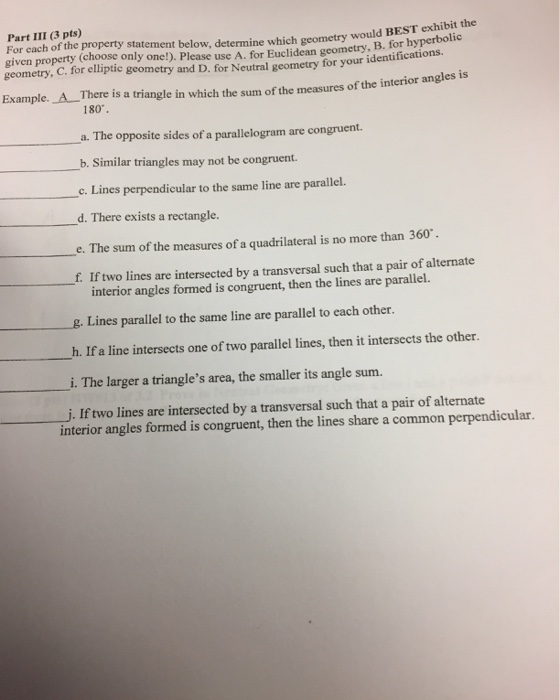Part III (3 pts) For cach of the property statement below, determine which geometry would BEST xhoi given property (choose only one!). Please use A. for Euclidean geometry, B. for hypere geometry, gcometry and D. for Neutral geometry for your identifications Example. A There is a triangle in which the sum of the measures of the interior angles is 180. a. The opposite sides of a parallelogram are congruent. b. Similar triangles may not be congruent. Lines perpendicular to the...

• ### Part II. (4 pts) Given the axiom set for the Incidence Geometry as below: Undefined terms: point, line, on Definitions: 1. Two lines are intersecting if there is a point on both. 2. Two lines are...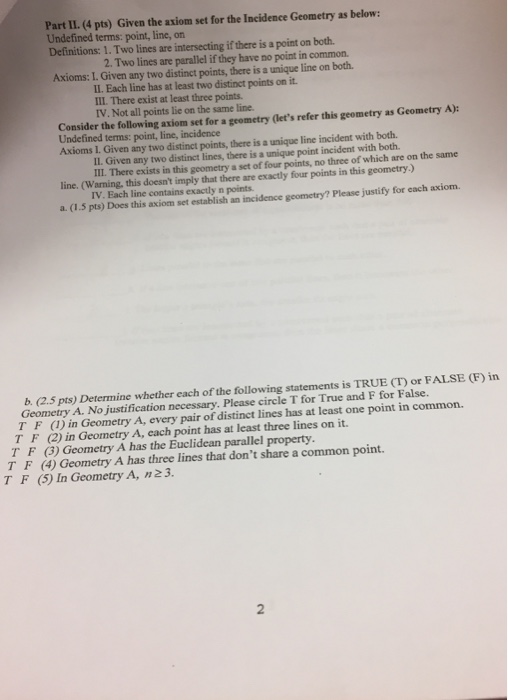Part II. (4 pts) Given the axiom set for the Incidence Geometry as below: Undefined terms: point, line, on Definitions: 1. Two lines are intersecting if there is a point on both. 2. Two lines are parallel if they have no point in common. Axioms: I. Given any two distinct points, there is a unique line on both. II. Each line has at least two distinct points on it. III. There exist at least three points. IV. Not all points...

• ### using these axioms prove proof number 5 1 - . Axiom 1: There exist at least...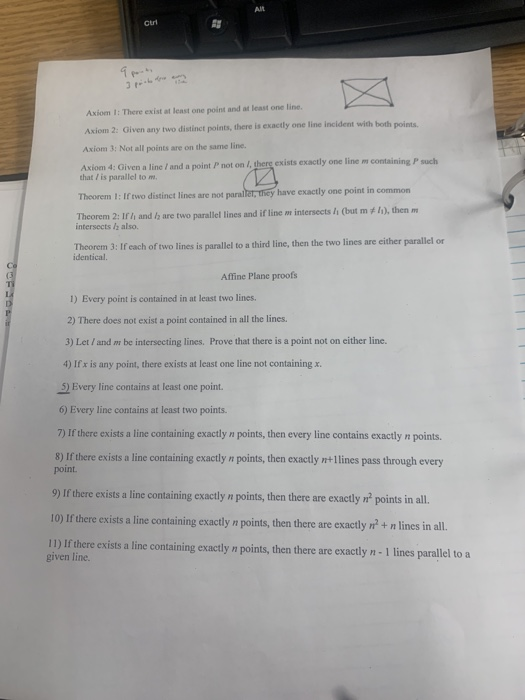using these axioms prove proof number 5 1 - . Axiom 1: There exist at least one point and at least one line Axiom 2: Given any two distinct points, there is exactly one line incident with both points Axiom 3: Not all points are on the same line. Axiom 4: Given a line and a point not on/ there exists exactly one linem containing Pouch that / is parallel tom Theorem 1: If two distinct lines are not parallet,...